• 微信小程序搜索功能，最近一直在做微信小程序的东西，所以整理一个搜索功能
• 主要介绍了微信小程序搜索功能(附：小程序前端+PHP后端)，小编觉得挺不错的，现在分享给大家，也给大家做个参考。一起跟随小编过来看看吧
• 微信小程序搜索功能实现步骤 先建好一个搜索词权重表，里面含有id、搜索词、权重(用数字来写) 微信小程序前台有传入搜索词到后台api，比如现在的sousuoa() 引入搜索词库，然后进行分词 获取分词后判断分词是否存在...
微信小程序api后台搜索的代码
微信小程序搜索功能实现步骤

先建好一个搜索词权重表，里面含有id、搜索词、权重(用数字来写)
微信小程序前台有传入搜索词到后台api，比如现在的sousuoa()
引入搜索词库，然后进行分词
获取分词后判断分词是否存在搜索词权重表里面不，如果存在然后处理那个分词权重大哪个就排在前面，最后执行sql语句，sql语句要有根据分词来排序，怎样排序根据个人功能来实现

       //搜索功能实现
public function sousuoa(){

$post=input();$uid=$_POST['uid']; //用户的id$urls=$_SERVER['HTTP_HOST'];//当前的域名$list=$_POST['list'];$pages=$_POST['pages'];//当前第几页 if($pages==0)
{
$pages_index=$pages;
}
else{
$pages_index=$pages*12;//一个页查的总数
}
$goods_discount=1; //默认第一张图,根据用户身份来显示第几张图片作为商品主图$number_pic=0;

if($uid){$member=Db::name('ns_member')->where('uid',$uid)->select(); if($member['stauts']==2){//判断用户是否认证
$member2=Db::name('ns_member_level')->where('level_id',$member['member_level'])->select();
$number_pic=$member2['zhutu'];//根据用户的身份来获取不同身份图片id集
$goods_discount=$member2['goods_discount'];//根据用户的身份来获取打几折
}
}
$data=trim($post['inputValue']);//过滤传过来的搜索词
include 'data/extend/PHPanalysis/phpanalysis.php';//引入搜索词库

$str = trim($data);
$do_fork = true;$do_unit = true;//新词识别
$do_multi = true;//多元切分$do_prop = false;//词性标注
$pri_dict = false;//是否预载全部词条$pa = new \PhpAnalysis('utf-8', 'utf-8', $pri_dict); //载入词典$pa->LoadDict();
//执行分词
$pa->SetSource($str);
$pa->differMax =$do_multi;
$pa->unitWord =$do_unit;
$pa->StartAnalysis($do_fork );
$str =$pa->GetFinallyResult(' ', $do_prop);$str = explode(' ',$str);$args = array();
foreach ($str as$key =>$v){ if(trim($v)=='' || trim($v)==')' || trim($v)=='(')continue;
$args[] =$v;
}

//搜索词权重
$search=[];$search_content=Db::name("nc_search")->select();//获取所有搜索词和权重
foreach ($search_content as$k=>$v) {$search[]=$v['keywords'].'-'.$v['number'];//把搜索词和权重组在一起

}
$search_content=[]; //遍历用户输入的搜索词由词库分词的数组，如何判断分词的词是否在建好搜索词权重表里面 foreach ($args as $key =>$value) {

foreach($search as$k=>$v ){ if (strstr($v , $value ) !== false ){//判断分词的词是否在建好搜索词权重表里面$number = substr($v,strripos($v,"-")+1);//截取"-"后面的内容，就是权重，用来排序作用
$search_content[$value]=$number;//把权重做值，搜索词做键名，避免重复 } } } arsort($search_content);//数组根据值来降序排序

$search_arr=[]; foreach ($search_content as $k1=>$v1){
$search_arr[]=$k1;//重新组成一维数组
}

$end = ""; foreach ($search_arr as $key=>$value)
{
$end =$key +1;
if($key == 0){$where = "where goods_name like '%$value%'";//模糊搜索条件$sql = "select * from ns_goods  %s% order by (case when goods_name = '%$value%' then$end ";//模糊排序
}else{
$where .= " or goods_name like '%$value%'";//模糊搜索条件
$sql .= " when goods_name like '%$value%' then $end"; } }$sql = $sql." end ) LIMIT$pages_index,12";//搜索sql的语句
$rsql = str_replace("%s%",$where, $sql);//模糊搜索条件组合替换 %s%$result = Db ::query($rsql);//执行sql的语句 //根据用户不同身份显示不同商品主图 foreach($result as $kk=>$vv){

$pic_arr=$vv['img_id_array'];//获取商品所有图片集
$pic_arr_list=explode(',',$pic_arr);//转换成数组
$number=count($pic_arr_list);//获取图片数组总数
if($number==1){$goods_pic_id=$pic_arr_list;//如果只有一个就获取第一个 }else{ if($number_pic==0){
$goods_pic_id=$pic_arr_list;
}else{
if($number_pic<$number){
$number=$number_pic-1;
$goods_pic_id=$pic_arr_list[$number]; }else{$goods_pic_id=$pic_arr_list; } } }$goodspic=Db::name('sys_album_picture')->where('pic_id',$goods_pic_id)->select();//根据用户不同身份显示不同商品主图$goods_thumb=$goodspic['pic_cover'];$result[$kk]['pic']="https://$urls/".$goods_thumb;$result[$kk]['goods_name']=mb_substr($vv['goods_name'],0,13,'utf-8');
$result[$kk]['price']= floor($vv['price']*$goods_discount);//打折后的价格取整

}

echo json_encode(result);//返回json格式给微信小程序前端 exit(); }   展开全文• 微信小程序已经是非常火了，而且学习也比较容易，但是对于初学者来说还是一件比较伤脑筋的事，接下来给大家分享一下小程序搜索的思路。 流程 1、表单（输入框、提交按钮、提交的name值) 2、接收表单数据（js获取... 开发需求 微信小程序已经是非常火了，而且学习也比较容易，但是对于初学者来说还是一件比较伤脑筋的事，接下来给大家分享一下小程序搜索的思路。 流程 1、表单（输入框、提交按钮、提交的name值) 2、接收表单数据（js获取表单name=keyword的值） 3、通过wx.request向服务器后端发起请求查询数据库 4、返回JSON格式的数据给小程序，js解析渲染到小程序前端展示 界面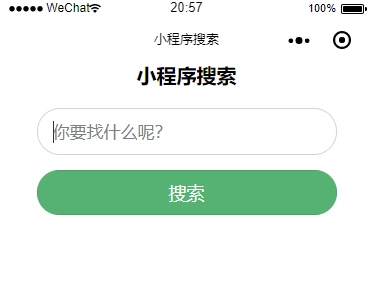代码 index.wxml <!-- 标题 --> <view class="title">小程序搜索</view> <!-- 搜索框view --> <view class="search_con"> <!-- 表单 --> <form bindsubmit="formSubmit"> <!-- 记得设置name值，这样JS才能接收name=keyword的值 --> <input type="text" name="keyword" class="search_input" placeholder='你要找什么呢？'/> <button formType="submit" class="search_btn">搜索</button> </form> </view> <!-- 搜索结果展示 --> <view wx:for="{{re}}" wx:key="re" class="search_result"> <!-- 当提交空白表单的时候 --> <view class="empty">{{item.empty}}</view> <!-- 当有搜索结果的时候 --> <view class="resname">{{item.resname}}</view> <!-- 当查询不到结果的时候 --> <view class="noresult">{{item.noresult}}</view> </view> index.js 其中里面的http://localhost/search.php?keyword= 是服务器后端接口，用于接收小程序传过去的关键词的，下面会有这个后端PHP文件。 const app = getApp() Page({ data: {}, //执行点击事件 formSubmit: function (e) { //声明当天执行的 var that = this; //获取表单所有name=keyword的值 var formData = e.detail.value.keyword; //显示搜索中的提示 wx.showLoading({ title: '搜索中', icon: 'loading' }) //向搜索后端服务器发起请求 wx.request({ //URL url: 'http://localhost/search.php?keyword=' + formData, //发送的数据 data: formData, //请求的数据时JSON格式 header: { 'Content-Type':'application/json' }, //请求成功 success: function (res) { //控制台打印（开发调试用） console.log(res.data) //把所有结果存进一个名为re的数组 that.setData({ re: res.data, }) //搜索成功后，隐藏搜索中的提示 wx.hideLoading(); } }) }, }) index.wxss /* 搜索样式 */ .title{ text-align: center; font-size: 20px; font-weight: bold; } .search_con{ width: 80%; margin:20px auto; } .search_con .search_input{ border: 1px solid rgb(214, 211, 211); height: 45px; border-radius: 100px; font-size: 17px; padding-left: 15px;/*此处要用padding-left才可以把光标往右移动15像素，不可以用text-indent*/ color: #333; } .search_con .search_btn{ margin-top: 15px; width: 100%; height: 45px; background: #56b273; color: #fff; border-radius: 100px; } .search_result{ width: 80%; margin:10px auto; } .search_result .empty{ text-align: center; color: #f00; font-size: 15px; } .search_result .noresult{ text-align: center; color: #666; font-size: 15px; } .search_result .resname{ text-align: left; color: #333; font-size: 15px; } 服务端 search.php <?php header('Content-Type:application/json'); //获取表单数据keyword1 = $_GET["keyword"]; //过滤表单空格$keyword2 = trim($keyword1); //当表单提交空白数据时 if(empty($keyword2)){

//构建数组
$arr = array( "empty" => "表单不能为空" ); //把数组转换为json$data = json_encode($arr); echo "[$data]";

}else{

//过滤表单特殊字符
$replace = array('!','@','#','$','%','^','&','*','(',')','_','-','+','=','{','}','[',']',';',':','"','<','>','?','/','|');
$keyword3 = str_replace($replace, '', $keyword2); // 连接数据库$con = mysql_connect("数据库地址","数据库账号","数据库密码");
if (!$con){die('Could not connect: ' . mysql_error());} mysql_select_db("数据库名",$con);
mysql_query("SET NAMES UTF8");

//查询数据库
$result = mysql_query("SELECT * FROM 表名 WHERE 需要查询的字段 like '%$keyword3%' ORDER BY ID DESC");
$results = array(); //查询数据库是否存在这条记录$exist = mysql_num_rows($result); if ($exist) {
//遍历输出
while ($row = mysql_fetch_assoc($result)){
$results[] =$row;
}

//输出JSON
echo json_encode($results); //当查询无结果的时候 }else{ //构建数组$arr = array(
"noresult" => "暂无结果"
);

//把数组转换为json
$data = json_encode($arr);
echo "[$data]"; } //断开数据库连接 mysql_close($con);
}
?>

服务端也是非常简单的，大家自己把服务端写好一点，毕竟安全和效率是很重要的。

演示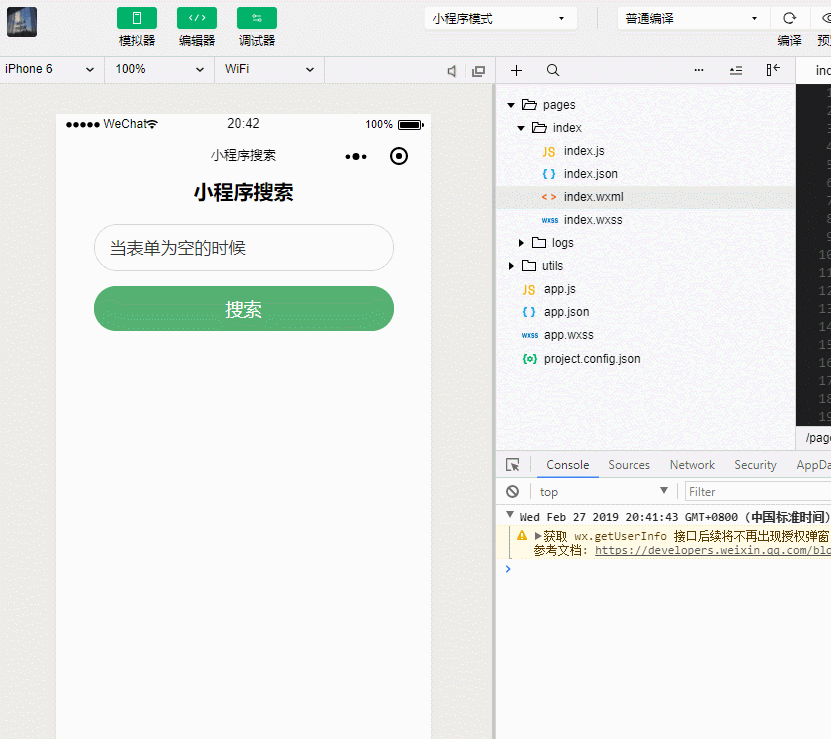作者：TANKING

网站：http://likeyunba.com

学习交流微信：face6009

（学习交流可以加我）

另外，本人喜欢研究支付，如果想要了解支付接口，微信支付，支付宝的个人免签约支付接口方案，可以看看我这个：

http://www.likeyunba.com/pay/

展开全文php 搜索
• 正在做一个微信小程序，想添加一个搜索功能，该怎么实现呢？搜索的内容要放到数据库里吗？最好有个案例学习学习。
• 小程序的pages里面的文件夹一样，放在和pages同一级用来给page的页面调用。
•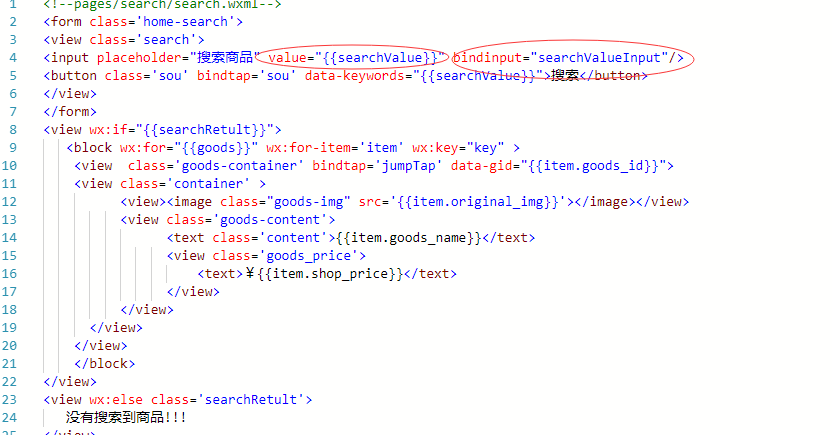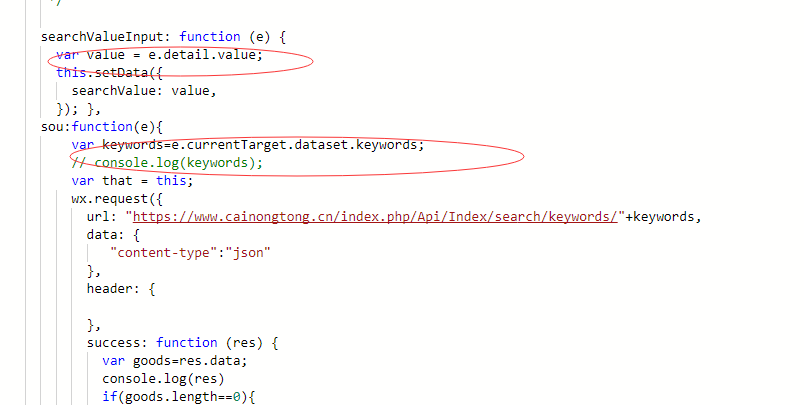展开全文• 现在微信小程序有重新推出新的功能，那就是进行模糊搜索，但是很多朋友微信小程序没有模糊搜索功能怎么办，下文小编就为大家带来详细介绍，一起看看吧现在微信小程序有重新推出新的功能，那就是进行模糊搜索，用户...
现在微信小程序有重新推出新的功能，那就是进行模糊搜索，但是很多朋友微信小程序没有模糊搜索功能怎么办，下文小编就为大家带来详细介绍，一起看看吧现在微信小程序有重新推出新的功能，那就是进行模糊搜索，用户不用非要进行准确的搜索。但是还有的小伙伴发现自己并没有模糊搜素这个功能，因此就让小编给大家详细的讲讲吧。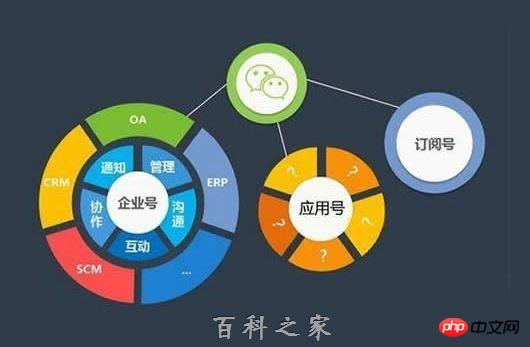微信小程序没有模糊搜索功能怎么回事由于设定于线下场景扫描进入，小程序没有任何集中入口，用户要想获得，只能扫码或者精确搜索完整的小程序名称(极少部分可以模糊搜索)，而由于小程序和公众号不能重名，所以名字一般都很长很复杂，搜索并不容易。今天，微信小程序全然上线了模糊搜索功能，直接搜索任意关键字，就会返回包含它的所有相关小程序。初步测试发现，美食、表情、音乐、电影、资讯、天气等常用关键字都已经开放，尤其是生活类的，但也不是所有关键字搜索都能得到相关小程序，比如搜索科技就没有任何结果。另外，微信版本必须是最新的6.5.4。以上就是本文的全部内容，希望对大家的学习有所帮助，更多相关内容请关注PHP中文网！相关推荐：
展开全文• 分享小程序最常见的搜索功能。后台基于PHP。后台框架TP5.1 1.后台框架搭建。composer安装TP5.1。 在 Windows 中，你需要下载并运行Composer-Setup.exe 打开命令行输入composer，如下图：表示安装成功 打开...PHP
• wx-gesture-lock 微信小程序的手势...WeixinAppBdNovel 微信小程序demo：百度小说搜索 shitoujiandaobu 小程序：石头剪刀布（附代码说明） audiodemo 微信小程序开发之视频播放器 Video 弹幕 弹幕颜色自定义
• 主要介绍了微信小程序实现搜索功能,本文通过实例代码给大家介绍的非常详细，对大家的学习或工作具有一定的参考借鉴价值，需要的朋友可以参考下
• 微信小程序开发交流qq群 173683895 承接微信小程序开发。扫码加微信。 正文： 上效果图: 一:搜索功能实现 1.在首页做一个搜索框的样式并实现跳转到搜索页面 <view class='page_row' bindtap=...
• 主要介绍了微信小程序搜索框样式并实现跳转到搜索页面(小程序搜索功能),需要的朋友可以参考下
• WeixinAppBdNovel微信小程序demo：百度小说搜索 shitoujiandaobu小程序：石头剪刀布（附代码说明） audiodemo微信小程序开发之视频播放器 Video 弹幕 弹幕颜色自定义 star微信小程序开发之五星评分 switchCity...
• 微信小程序入门与实战 第1章 什么是微信小程序 第2章 小程序环境搭建与开发工具介绍 第3章 第一个简单的页面：欢迎页面 第4章 第二个页面：新闻阅读页面 第5章 小程序的模板化与模块儿化 第6章 构建新闻详情页面 第7...
• 微信小程序语音搜索功能 功能简介：微信小程序实现语音搜索（模仿微信发送语音功能）按住按钮说话，松开按钮自动识别 主要用到的技术： 1.微信录音api 2.后台调用百度语音实现录音转化成文字返回前端 //...语音转文字
• 主要为大家详细介绍了微信小程序实现搜索历史功能，具有一定的参考价值，感兴趣的小伙伴们可以参考一下
• 主要介绍了微信小程序实现搜索功能并跳转搜索结果页面，具有一定的参考价值，感兴趣的小伙伴们可以参考一下
• 主要介绍了微信小程序下拉框搜索功能的实现方法，内容比较简单容易理解 ,需要的朋友可以参考下
• 前段时间做了一个微信小程序的项目，在项目中有实现过小程序的转发功能,这里给大家分享一下，有不对的地方勿喷 微信小程序官方文档:https://developers.weixin.qq.com/miniprogram/dev/api/ 实现小程序转发有二种...
• 微信小程序--健康菜谱 基于微信小程序的一个查找检索菜谱的应用 效果 功能 分类检索菜谱 发现最新菜谱 根据菜谱名搜索菜谱 用到的API 页面路由 列表渲染 带参数页面跳转 navigator scrollview下拉加载更多 ... ...
• 微信小程序版豆瓣电影 概述 微信小程序版豆瓣电影（非官方出品） 数据来源：豆瓣电影API 开发工具：微信开发者工具 0.14.140900 功能 电影榜单列表 电影搜索 电影条目信息 影人条目信息 预览 其他 声明：非豆瓣...
• 为丰富小程序的服务能力，提高服务质量，微信小程序提供客服消息能力，以便小程序用户可以方便快捷地与小程序服务提供方进行沟通。 功能介绍 用户可使用小程序客服消息功能，与小程序的客服人员进行沟通。 客服...
• WeixinAppBdNovel 微信小程序demo：百度小说搜索 shitoujiandaobu 小程序：石头剪刀布（附代码说明） audiodemo 微信小程序开发之视频播放器 Video 弹幕 弹幕颜色自定义 star 微信小程序开发之五星评分 swit...
• 主要为大家详细介绍了微信小程序实现日历功能，具有一定的参考价值，感兴趣的小伙伴们可以参考一下
• 一款基于微信小程序开发的网络用语科普与查询工具，是本人先前《网络用语词典》作品的微信小程序版本。 使用方法 Usage 克隆项目仓库 \$ git clone https://github.com/anegie/wxa-internet-slangs.git 导入微信 ...
• PP商城，开源商城（微信小程序端） 基于开源项目NideShop重建，精简了一些功能的同时完善了一些功能，并重新设计了UI 测试数据来自上述开源项目 服务端api基于Ｎode.js+ThinkJS+MySQL 目前基于PP商城已经上线的微信...
• 微信小程序大作业，里面有十个项目，有微信商城小程序，微信播放器小程序，微信旅游小程序，微信电影小程序等等，都适合初学者学习，都有登录（登录账号和密码为abc和123），轮播图，导航栏，部分有搜索功能...

# 微信小程序搜索功能微信小程序 订阅